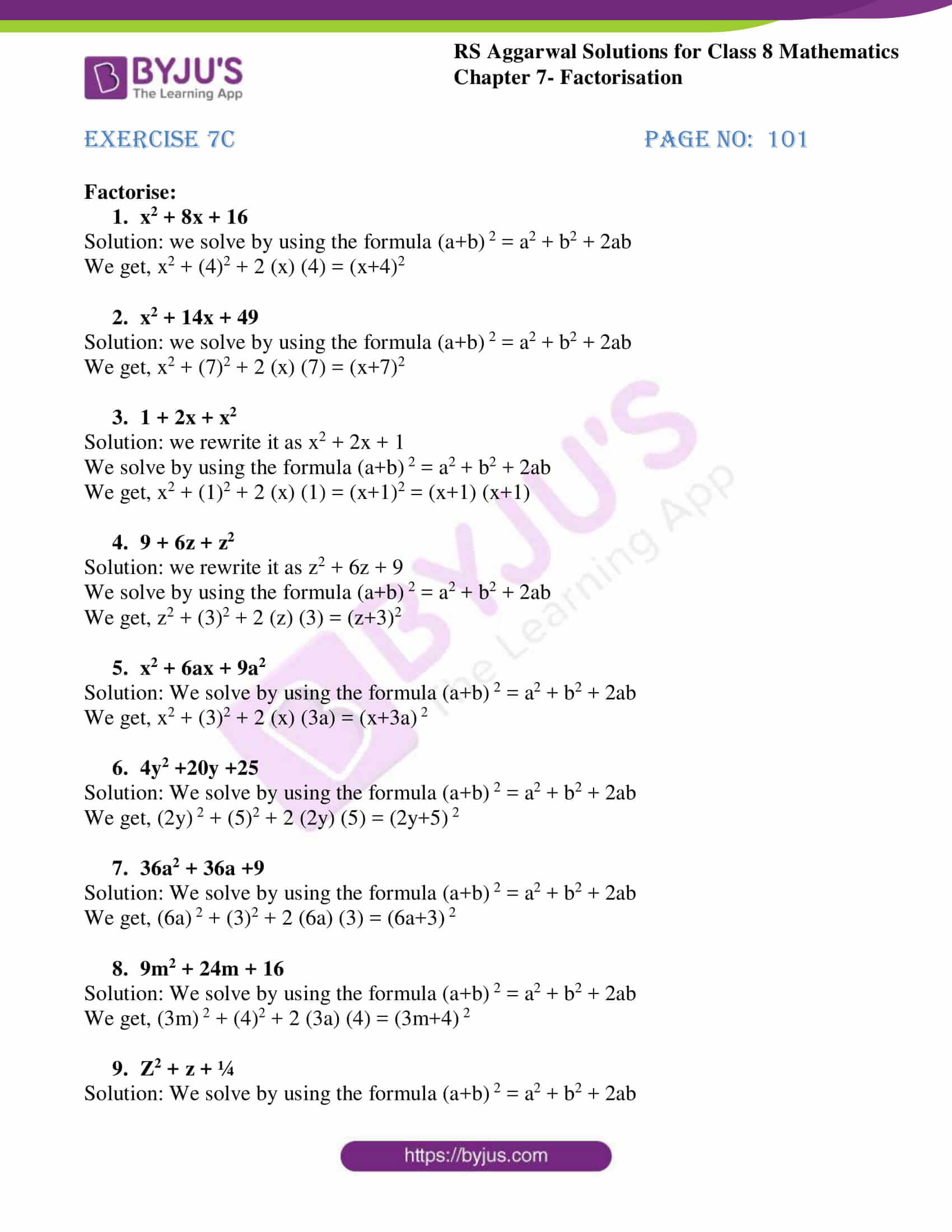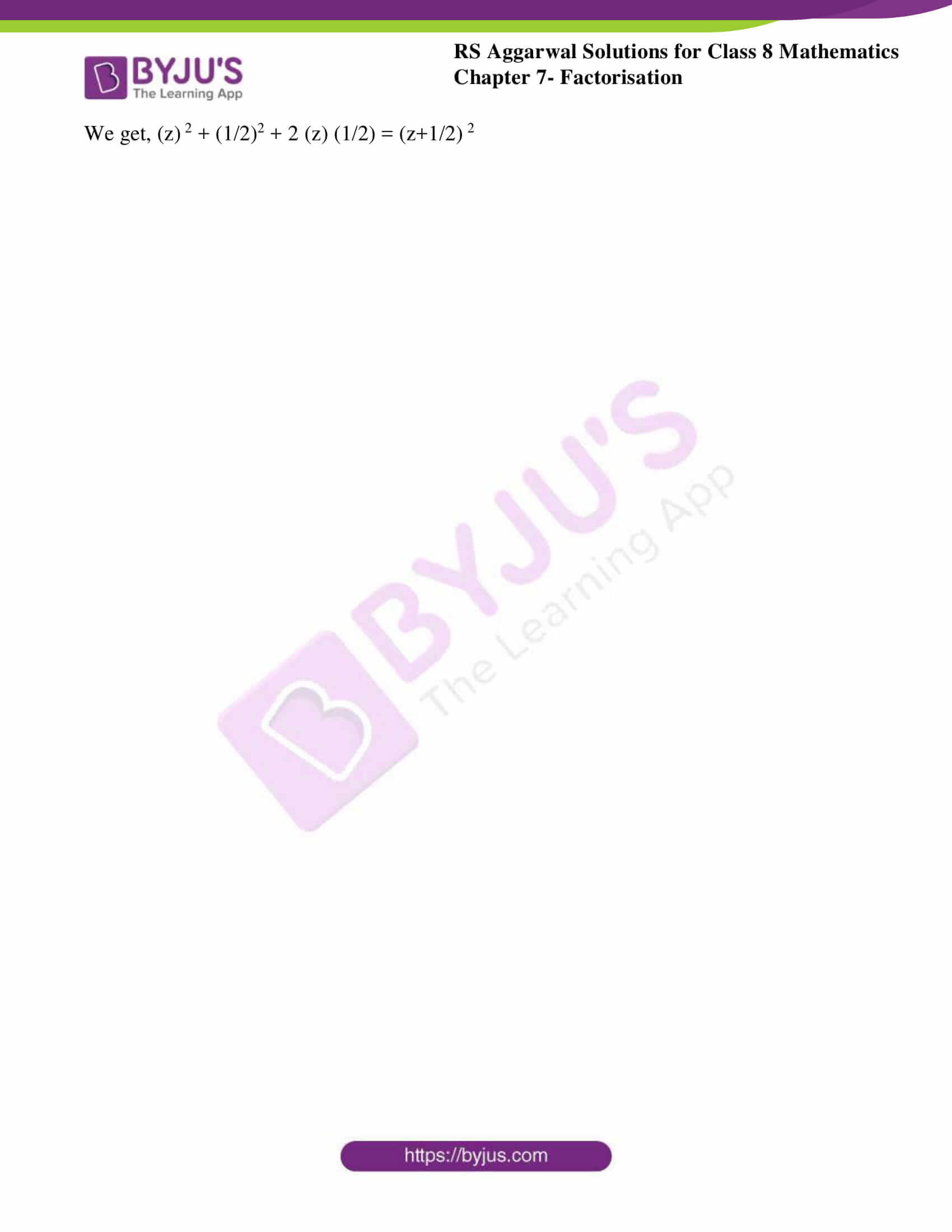# RS Aggarwal Solutions for Class 8 Chapter 7 - Factorisation Exercise 7C

RS Aggarwal Solutions for Class 8 Maths Chapter 7- Exercise 7C, Factorisation links are provided below, students can refer and download them. Our experts have solved the RS Aggarwal Solutions to ensure that the students are precise with their basic concepts by practicing the solutions. In Exercise 7C of RS Aggarwal Class 8 Maths, we shall study one of the methods i.e. Factorisation when a given expression is a perfect square.

## Download PDF of RS Aggarwal Solutions for Class 8 Maths Chapter 7- Exercise 7C### Access Answers to RS Aggarwal Solutions for Class 8 Maths Chapter 7- Factorisation Exercise 7C

Factorise:

1. x2 + 8x + 16

Solution: we solve by using the formula (a+b) 2 = a2 + b2 + 2ab

We get, x2 + (4)2 + 2 (x) (4) = (x+4)2

2. x2 + 14x + 49

Solution: we solve by using the formula (a+b) 2 = a2 + b2 + 2ab

We get, x2 + (7)2 + 2 (x) (7) = (x+7)2

3. 1 + 2x + x2

Solution: we rewrite it as x2 + 2x + 1

We solve by using the formula (a+b) 2 = a2 + b2 + 2ab

We get, x2 + (1)2 + 2 (x) (1) = (x+1)2 = (x+1) (x+1)

4. 9 + 6z + z2

Solution: we rewrite it as z2 + 6z + 9

We solve by using the formula (a+b) 2 = a2 + b2 + 2ab

We get, z2 + (3)2 + 2 (z) (3) = (z+3)2

5. x2 + 6ax + 9a2

Solution: We solve by using the formula (a+b) 2 = a2 + b2 + 2ab

We get, x2 + (3)2 + 2 (x) (3a) = (x+3a) 2

6. 4y2 +20y +25

Solution: We solve by using the formula (a+b) 2 = a2 + b2 + 2ab

We get, (2y) 2 + (5)2 + 2 (2y) (5) = (2y+5) 2

7. 36a2 + 36a +9

Solution: We solve by using the formula (a+b) 2 = a2 + b2 + 2ab

We get, (6a) 2 + (3)2 + 2 (6a) (3) = (6a+3) 2

8. 9m2 + 24m + 16

Solution: We solve by using the formula (a+b) 2 = a2 + b2 + 2ab

We get, (3m) 2 + (4)2 + 2 (3a) (4) = (3m+4) 2

9. z2 + z + ¼

Solution: We solve by using the formula (a+b) 2 = a2 + b2 + 2ab

We get, (z) 2 + (1/2)2 + 2 (z) (1/2) = (z+1/2) 2

## RS Aggarwal Solutions for Class 8 Maths Chapter 7- Exercise 7C

Exercise 7C of RS Aggarwal Class 8, Factorisation. This exercise mainly deals with Factorising when a given expression is a perfect square. The RS Aggarwal Solutions can help the students practice attentively while learning the fundamentals as it provides all the answers to the questions from the RS Aggarwal textbook. Students are suggested to practice the problems on a regular basis which will help them ace the exams and increase their overall percentage. Practising as many times as possible helps in building time management skills and also boosts the confidence level to achieve high marks.